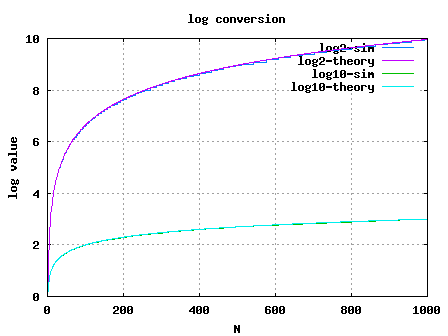- DSP log - http://www.dsplog.com -

Linear to log conversion

Posted By Krishna Sankar On November 20, 2008 @ 6:40 am In DSP | 10 Comments

In signal processing blocks like power estimation used in digital communication, it may be required to represent the estimate in log scale. This post explains a simple linear to log conversion scheme proposed in the DSP Guru column on DSP Trick: Quick-and-Dirty Logarithms. The scheme makes implementation of a linear to log conversion simple and small in a digital hardware like FPGA.

Consider an integer . The floating point representation is,

where,

is the exponent and

is the mantissa.

Assume that is normalized, i.e .

Taking logarithm to the base 2,

.

In digital hardware implementations, finding the exponent is simple. Its just noting the index of the first bit which is 1 starting from MSB side.

For example consider an input number .

Expressed in binary on 8 bit bus, .

The value of in this example is 3.

Now, the part which remains to be computed is the mantissa . In this example,

.

Given that lies in the range . this can be computed using a Look Up Table. The LUT can store values of input between 1 to 2. The precision requirement determines the number of elements in the LUT. Let us assume that we want to have a precision of , where . The look up table values will be as follows:

 k=4 index. j Linear = 1+j/2^k LUT = log_2(Linear) 1 1.06250 0.0874628 2 1.12500 0.1699250 3 1.18750 0.2479275 4 1.25000 0.3219281 5 1.31250 0.3923174 6 1.37500 0.4594316 7 1.43750 0.5235620 8 1.50000 0.5849625 9 1.56250 0.6438562 10 1.62500 0.7004397 11 1.68750 0.7548875 12 1.75000 0.8073549 13 1.81250 0.8579810 14 1.87500 0.9068906 15 1.93750 0.9541963 16 2.00000 1.0000000

Table: Look up table values for logarithm computation

From the above look up table, we can see that mantissa of corresponds to index of . It is inituitive to note that the array index can be found out by the simple formula,

. To handle cases where this number can can be a fraction, the result is floored to the nearest integer, i.e.

So the value of in base is,

.Once we have the number in base, conversion to any other base is simple.

.

So the number in base is,

.

## Simulation Model

Simple Matlab/Octave script for computing the logarithm via the LUT based approach is provided. Click here to download Matlab/Octave script for performing linear to log conversion using LUT based approachFigure: Linear to log conversion using LUT